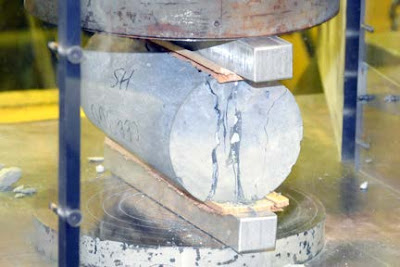## 14 Feb 2019

### Concrete and Its PropertiesNormal Concrete

## Concrete

Concrete is the mixture of Coarse aggregate, Fine aggregate, Water, Cement, Air (entraped) and sometimes admixtures.

### Proportions of the Materials

The normal composition of concrete include
• Cement→ 7% to 15%
• Aggregate (Fine and Coarse Aggregate)→ 60% to 75%
• Water→ 14% to 21%
• Air (entraped)→ 4% to 8%

## Properties of Concrete

### Compressive Strength

The uniaxial compressive strength is normally measured by compressive test of a standard cylinder, normally tested after 28 days of curing. Using the testing method of ASTM standard "C31" and 'C39". The compressive strength of concrete is normally represented by strain-stress curve. Which is used to check concrete strength for quality control. The normal rang for standard concrete strength is:

fc'= 3000 psi to fc'= 5000 psi

fc'= Compressive strengthConcrete Compressive Test

### Tensile Strength

This strength normally ranges from 8% to 15% of compressive strength (fc'). The type of test used to determine tensile strength is
1. Flexure Test
2. Split Cylinder TestConcrete Tensile Test

### Relationship b/w Compressive & Tensile Strength

The concrete tensile strength increases with increase in compressive strength. So these both properties are directly proportional to each other:

Tensile Strength ∝ √fc'Concrete